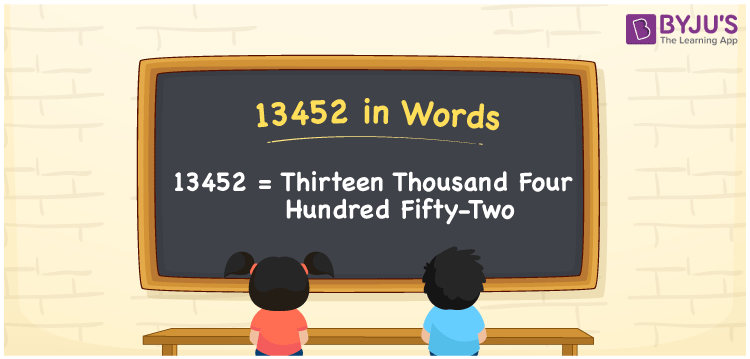# 13452 in Words

13452 in words can be written as Thirteen Thousand Four Hundred Fifty-two. The article of 13452 in words will provide students with a clear knowledge of the concept of counting numbers. If Rs. 13452 is spent on buying a new sofa set, then you can say that “I spent Thirteen Thousand Four Hundred Fifty-two Rupees in buying a new sofa set”. The  concept of converting 13452 into words using the English alphabet is discussed here. Students will be able to convert numbers in words with the help of the resources available at BYJU’S. 13452 can be read as “Thirteen Thousand Four Hundred Fifty-two” in English.

 13452 in words Thirteen Thousand Four Hundred Fifty-two Thirteen Thousand Four Hundred Fifty-two in Numbers 13452

## 13452 in English Words## How to Write 13452 in Words?

In this section you will learn in detail about conversion of 13452 into words using the place value chart. Five digits are present in 13452. The place value chart of 13452 can be understood easily using the table below.

 Ten Thousands Thousands Hundreds Tens Ones 1 3 4 5 2

The expanded form of the number 13452 is given below:

1 x Ten Thousand + 3 × Thousand + 4 × Hundred + 5 × Ten + 2 × One

= 1 x 10000 + 3 × 1000  + 4 × 100 + 5 × 10 + 2 × 1

= 10000 + 3000 + 400 + 50 + 2

= 13452

= Thirteen Thousand Four Hundred Fifty-two

Hence, 13452 in words is written as Thirteen Thousand Four Hundred Fifty-two.

13452 is a natural number that precedes 13453 and succeeds 13451.

13452 in words – Thirteen Thousand Four Hundred Fifty-two

Is 13452 an odd number? – No

Is 13452 an even number? – Yes

Is 13452 a perfect square number? – No

Is 13452 a perfect cube number? – No

Is 13452 a prime number? – No

Is 13452 a composite number? – Yes

## Frequently Asked Questions on 13452 in Words

Q1

### Write 13452 in words.

13452 can be written as “Thirteen Thousand Four Hundred Fifty-two” in words.
Q2

### Is 13452 an odd or even number?

13452 is an even number because it is divisible by 2.
13452/2 = 6726
Q3

### Write Thirteen Thousand Four Hundred Fifty-two in numbers.

Thirteen Thousand Four Hundred Fifty-two can be written in numbers as 13452.